# Excercise 6.4 The-Triangle-and-its-Properties- NCERT Solutions Class 7

## Chapter 6 Ex.6.4 Question 1

Is it possible to have a triangle with the following sides?

(i) $$\rm{}2\, cm,$$ $$\rm{}3\,cm,$$ $$\rm{}5\, cm$$

(ii) $$\rm{}3 \,cm, 6\, cm, 7\, cm$$

(iii) $$\rm{}6 \,cm, 3\, cm, 2\,cm$$

### Solution

What is known?

Sides of triangle.

What is unknown?

Is it possible to have a triangle with these sides.

Reasoning:

This problem is based on a very simple property that if the sum of lengths of any two sides of a triangle is greater than the third side, then it is possible to draw a triangle. By using this property, you just have to check by taking the sum of any two sides of a triangle whether it is greater than the third side or not. For better understanding take any two sides one by one and check if sum of these two sides is greater than the third side or not, if it is greater than the third sides then it is possible to draw a triangle.

(i) $$\rm{}2+3>5$$ No

$$\rm{}3+5>2$$ Yes

$$\rm{}5+2>3$$ Yes

No, the triangle is not possible

(ii)  $$\rm{}3+6>7$$  Yes

$$\rm{}6+7>3$$  Yes

$$7+3>3$$  Yes

Yes, the triangle is possible

(iii)  $$\rm{}6+3>2$$  Yes

$$\rm{} 3+2>6$$  No

$$\rm{}2+6>3$$  Yes

No, the triangle is not possible

Useful Tip

Whenever you encounter problems of this kind, it is best to think of the property based on sum of lengths of any two sides of a triangle.

## Chapter 6 Ex.6.4 Question 2

Take any point $$\rm{}O$$ in the interior of a triangle $$\rm{}PQR$$ Is

(i) $$\rm{}OP + OQ > PQ?$$

(ii) $$\rm{}OQ + OR > QR?$$

(iii) $$\rm{}OR + OP > RP?$$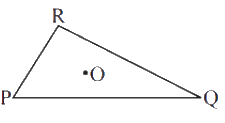### Solution

What is known?

Triangle $$PQR$$ and point in the interior of triangle.

What is unknown?

(i) $$OP + OQ > PQ?$$

(ii) $$OQ + OR > QR?$$

(iii) $$OR + OP > RP?$$

Reasoning:

This question is straight forward. If $$\rm{}“o”$$ is a point in the interior of a given triangle, then the three triangles can be constructed. You must join all the three vertices of a triangle with the centre $$\rm{}“o”$$. This question is based on a property that in a triangle the sum of lengths of any two sides is always greater than the third side.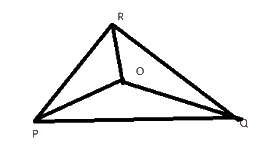Steps:

Yes, $$\rm{}POQ$$ forms a triangle

Yes, $$\rm{}OQR$$ forms a triangle.

Yes, $$\rm{}ORP$$ forms a triangle.

## Chapter 6 Ex.6.4 Question 3

$$AM$$ is a median of a triangle $$ABC.$$ Is $$AB + BC + CA > 2 AM?$$ (Consider the sides of triangles $$\Delta{ABM}$$ and $$\Delta{AMC}$$.)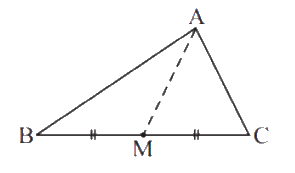### Solution

What is known?

$$ABC$$ is a triangle and $$AM$$ is a median of triangle $$ABC$$.

What is unknown?

Is $$AB + BC + CA > 2\, AM?$$

Reasoning:

In this question it is asked if $$AB + BC + CA > 2 AM$$ or not. This question is also based on the property that the sum of lengths of two sides of a triangle is always greater than the third side. In such kind of problems, you just visually identify the triangle $$ABC$$ and $$AM$$ is the median which further divides triangle $$ABC$$ into two more triangles i.e. triangle $$ABM$$ and $$AMC.$$ Now consider any two sides of first triangle $$ABM$$ and use the above property then consider the any two sides of another triangle $$AMC$$ and use the above property. Now, add $$\rm{}L.H.S.$$and $$\rm{}R.H.S.$$ of both the triangles.

Steps:

In triangle $$ABM,$$

$$AB + BM > AM{\rm{ }} \qquad \ldots .{\rm{ }}\left( 1 \right)$$

In triangle $$AMC,$$

$$AC + MC > AM{\rm{ }} \qquad \ldots .{\rm{ }}\left( 2 \right)$$

Adding equation $$\rm{}(1)$$ and $$\rm{}(2)$$ we get,

\begin{align}&AB \!+\! BM \!+\! AC \!+\! MC \!>\! AM \!+\! AM\\&AB \!+\! AC \!+\! BM \!+\! MC \!>\! 2AM\\&AB \!+\! AC \!+\! BC \!>\! 2AM\end{align}

Hence, it is true

Useful Tip:

Whenever you encounter problems of this kind, it is best to think of the property based on sum of lengths of any two sides of a triangle is always greater than the third side

## Chapter 6 Ex.6.4 Question 4

$$ABCD$$ is a quadrilateral. Is $$AB\! +\! BC\! +\! CD \!+\! DA\! >\! AC\! +\! BD?$$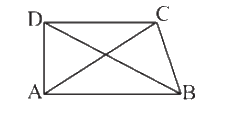### Solution

What is known?

$$ABCD$$ is a quadrilateral. $$DB$$ and $$AC$$ are diagonals.

What is unknown?

Is $$AB \!+\! BC\!+\!CD\!+\!DA \!>\! AC\!+\!BD?$$

Reasoning:

In this question, it is asked to check Is $$AB \!+\! BC \!+\! CD \!+\! DA \!>\! AC \!+\! BD$$or not. This question is based on the property that the sum of lengths of two sides of a triangle is always greater than the third side. Now visually identify that the quadrilateral $$ABCD$$ is divided by diagonals $$AC$$ and $$BD$$ into four triangles. Now, take each triangle separately and apply the above property and then add $$\rm{}L.H.S$$ and $$\rm{}R.H.S$$ of the equation formed.

Steps:

In triangle $$ABC,$$

$$AB + BC > AC{\rm{ }} \qquad \ldots \ldots .{\rm{ }}\left( 1 \right)$$

In triangle $$ADC,$$

$$AD + CD > AC{\rm{ }} \qquad \ldots \ldots .{\rm{ }}\left( 2 \right)$$

In triangle $$ADB,$$

$$AD + AB > DB{\rm{ }} \qquad \ldots \ldots .{\rm{ }}\left( 3 \right)$$

In triangle $$DCB,$$

$$DC + CB > DB{\rm{ }} \qquad \ldots \ldots .{\rm{ }}\left( 4 \right)$$

Adding equation $$\rm{}(1),$$ $$\rm{}(2),$$ $$\rm{}(3)$$ and $$\rm{}(4)$$ we get,

\begin{align}& \left[ \begin{array}& AB\!+\!BC\!+\!\\AD\!+\!CD\!+\!\\AD\!+\!AB\!+\! \\ DC\!+\!CB \\ \end{array} \right]\!>\!\left[ \begin{array}& AC\!+\!AC+\! \\ DB\!+\!DB \\ \end{array} \right] \\ & \left[ \begin{array} & AB\!+\!AB\!+\!BC\!+\! \\ BC\!+\!CD\!+\!CD\!+\! \\ AD\!+\!AD \\ \end{array} \right]\!>\!2AC\!+\!2DB \\ & \left[ \begin{array} & 2AB\!+\!2BC\!+\! \\ 2CD\!+\!2AD \\ \end{array} \right]\!>\!2AD\!+\!2DB \\ & AB\!+\!BC\!+\!CD\!+\!AD\!>\!AC\!+\!DB \\ \end{align}

Useful Tip:

Whenever you encounter problems of this kind, it is best to think of the property based on sum of lengths of any two sides of a triangle is always greater than the third side.

## Chapter 6 Ex.6.4 Question 5

$$ABCD$$ is quadrilateral. Is $$AB \!+\! BC \!+\! CD \!+\! DA\! <\! 2 (AC \!+\! BD)?$$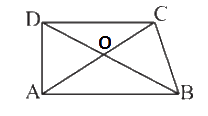### Solution

What is known?

$$ABCD$$ is a quadrilateral. $$DB$$ and $$AC$$ are diagonals.

What is unknown?

Is $$AB \!+\! BC \!+\! CD \!+\! DA \!<\! 2 (AC \!+\! BD)?$$

Reasoning:

In this question, it is asked to check Is $$AB \!+\! BC \!+\! CD \!+\! DA \!<\!2(AC \!+\! BD)$$ or not.
This question is based on the property that the sum of lengths of two sides of a triangle is always greater than the third side.$$“O”$$ is the centre of the quadrilateral.  Now visually identify that the quadrilateral $$ABCD$$ is divided by diagonals $$AC$$ and $$BD$$ into four triangles. Now, take each triangle separately i.e. triangle $$AOB, COD, BOC$$ and $$AOD$$ and apply the above property and then add $$L.H.S$$ and $$R.H.S$$ of the equation formed.

Steps:

In triangle $$AOB,$$

$$AB < OA + OB{\rm{ }} \qquad \ldots \ldots .{\rm{ }}\left( 1 \right)$$

In triangle $$COD,$$

$$CD < OC + OD{\rm{ }} \qquad \ldots \ldots .{\rm{ }}\left( 2 \right)$$

In triangle $$AOD,$$

$$DA < OD + OA{\rm{ }} \qquad \ldots \ldots .{\rm{ }}\left( 3 \right)$$

In triangle $$COB,$$

$$BC < OC + OB{\rm{ }} \qquad \ldots \ldots .{\rm{ }}\left( 4 \right)$$

Adding equation $$(1),$$ $$(2),$$ $$(3)$$ and $$(4)$$ we get,

\begin{align}& \left[ \begin{array}& AB\!+\!BC\!+\!\\CD\!+\!DA \end{array} \right]\!<\!\left[ \begin{array}& OA\!+\!OB\!+\! \\ OC\!+\!OD\!+\!\\OD\!+\!OA\!+\! \\ OC\!+\!OB \\ \end{array} \right] \\ =& \left[ \begin{array}& AB\!+\!BC\!+\!\\CD\!+\!DA\end{array} \right]<\left[ \begin{array}&2OA\!+\!2OB\!+\!\\2OC\!+\!2OD\end{array} \right] \\ =& \left[ \begin{array} & AB\!+\!BC\!+\! \\ CD\!+\!DA \\ \end{array} \right]<\left[ \begin{array}&2(OA\!+\!OB)\!+\!\\2(OC\!+\!OD)\end{array} \right] \\ = & AB\!+\!BC\!+\!CD\!+\!DA\!<\!2(AC\!+\!BD) \\ \end{align}

Yes, it is true.

Useful Tip

Whenever you encounter problems of this kind, it is best to think of the property based on sum of lengths of any two sides of a triangle is always greater than the third side.

## Chapter 6 Ex.6.4 Question 6

The lengths of two sides of a triangle are $$\rm{}12\, cm$$ and $$\rm{}15\,cm.$$ Between what two measures should the lengths of third side fall.

### Solution

What is known?

The lengths of two sides of a triangle are $$12$$ cm and $$15$$ cm.

What is unknown?

Between what two measures should the lengths of third side fall.

Reasoning:

This question is straight forward. you just have to remember one property that the sum of lengths of any two sides of a triangle is always greater than the third side and also the difference between the length of any two sides of a triangle is smaller than the length of third side. In this question, two sides of a triangle are given as $$\rm{}12\,cm$$ and $$\rm{}15\,cm$$, find the sum and difference of this two sides. Remember, the third side should be lesser than their sum and also it should be greater than their difference.

Steps:

We know that, Sum of lengths of any two sides of a triangle is always greater than the
third side and also the difference between the lengths of any two sides is always smaller than the third side.

Hence the third side will be lesser than the sum of these two sides $$\rm{}12\,cm + 15\,cm =27\, cm$$ and also it will be greater than the difference of these two sides $$\rm{}15\,cm – 12\,cm =3\,cm.$$ Therefore, length of third side will be smaller than $$\rm{}27\,cm$$ and greater than $$\rm{}3\,cm.$$

Instant doubt clearing with Cuemath Advanced Math Program